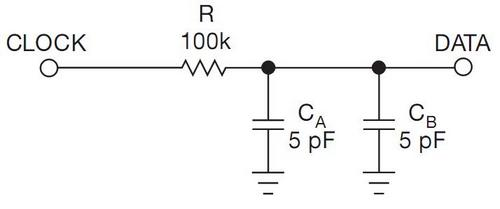# Drive 16 LEDs with one I/O line

Zoran Mijanovic and Nedjeljko Lekic, University of Montenegro, Podgorica, Montenegro

Use a shift register to successively turn on any LED.

Over the last few years, several Design Ideas have described how to use just a few microcontroller I/O pins to drive many LEDs (references 1 through 7). The circuit in Figure 1 can drive 16 LEDs with just one pin and two shift registers 74HC164. You can use the circuit to drive long-dot-bar or two seven-segment-digit displays. Adding multiplexing to the same circuit enables it to drive eight seven-segment LED digits.

 Figure 1. A 16-LED dot-bar/bar-graph display uses two 8-bit serial-input/parallel-output shift registers.

The microcontroller ATtiny13 drives the shift registers’ clock inputs. That signal also passes through an RC filter and drives data inputs A and B. A 100-kΩ resistor, R, and the A and B input pins’ capacitances form the RC filter (Figure 2), producing time delay of approximately R×C×ln2=100 kΩ×(5 pF+5 pF)×0.7=0.7 μsec.Figure 2. An RC filter provides a 0.7-μsec delay.

To write a logic zero to the shift register, the microcontroller holds a low level for approximately 2 μsec, which is longer than the time delay. It then sets the signal to a logic one, or high, level. To write a logic one, the microcontroller holds the high level for longer than the time delay. The microcontroller then makes negative pulses of approximately 0.25 μsec, or two CPU cycles, which is shorter than the time delay and which doesn’t change the logic level at the data inputs.

Figure 3 shows the clock signal in Channel 1 (yellow) and the data signal in Channel 2 (blue). The oscilloscope is a Tektronix DPO4034 with TPP0850 high-voltage probes. These probes have 40-MΩ input resistance and only 1.5-pF input capacitance, minimizing distortion.

 Figure 3. The waveform shows the circuit writing the pattern 1111111111000000 for the display. The upper, yellow trace is the clock signal, and the lower, blue trace is the data signal.

Drive 16 LEDs with one I/O line figure 3A rising edge on the clock signal clocks the shift registers. This edge corresponds to the data signal’s local minimum. Figure 3 also shows that the minimum data-signal voltages for logic zero and logic one are 1.3 and 3.1V, respectively. The shift register’s logical threshold is 2.5V. These voltages guarantee sufficient voltage margins. If your design requires higher margins, vary the signal timing and use a higher resistance for R in Figure 1. This circuit stores 16 bits in shift registers in approximately 35 μsec.

You can view a short video of the circuit in operation here and download a code listing, in C here. The software turns on the LEDs one by one every 500 msec until all LEDs are on. It then turns off all the LEDs and repeats the cycle.

Demonstration Video

EDN